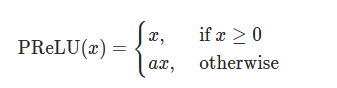# How can I incorporate PReLU in a quantized model?

Hello everyone.
This is a followup question concerning this . The issue is in the Resnet model that I’m dealing with, I cant replace `PReLU` with `ReLU` as it drastically affects the network performance.
So my question is, what are my options here? what should I be doing in this case?
Would doing sth like this suffice?

``````class PReLU_Quantized(nn.Module):
def __init__(self, prelu_object):
super().__init__()
self.weight = prelu_object.weight
self.quantized_op = nn.quantized.FloatFunctional()
self.quant = torch.quantization.QuantStub()
self.dequant = torch.quantization.DeQuantStub()

def forward(self, inputs):
# inputs = torch.max(0, inputs) + self.weight * torch.min(0, inputs)
self.weight = self.quant(self.weight)
weight_min_res = self.quantized_op.mul(self.weight, torch.min(inputs))
self.weight = self.dequant(self.weight)
return inputs
``````

and for the replacement :

``````class model(nn.Module):
def __init__(self)
super().__init__()
....
self.prelu = PReLU()
self.prelu_q = PReLU_Quantized(self.prelu)
....
``````

for some reason, the error between the actual PReLU and my implementation is very large!
here are sample diffs in different layers:

``````diff : 1.1562038660049438
diff : 0.02868632599711418
diff : 0.3653906583786011
diff : 1.6100226640701294
diff : 0.8999372720718384
diff : 0.03773299604654312
diff : -0.5090572834014893
diff : 0.1654307246208191
diff : 1.161868691444397
diff : 0.026089997962117195
diff : 0.4205571115016937
diff : 1.5337920188903809
diff : 0.8799554705619812
diff : 0.03827812895178795
diff : -0.40296515822410583
diff : 0.15618863701820374
``````

and the diff is calculated like this in the forward pass:

``````    def forward(self, x):
residual = x
out = self.bn0(x)
out = self.conv1(out)
out = self.bn1(out)
out = self.prelu(out)

out2 = self.prelu2(out)
print(f'diff : {( out - out2).mean().item()}')

out = self.conv2(out)
``````

This is the normal implementation which I used on ordinary model (i.e. not quantized!) to assess whether it produces correct result and then move on to quantized version:

``````class PReLU_2(nn.Module):
def __init__(self, prelu_object):
super().__init__()
self.prelu_weight = prelu_object.weight
self.weight = self.prelu_weight

def forward(self, inputs):
x = self.weight
tmin, _ = torch.min(inputs,dim=0)
tmax, _ = torch.max(inputs,dim=0)
weight_min_res = torch.mul(x, tmin)
inputs = inputs.unsqueeze(0)
return inputs

``````

what am I missing here?

OK, I figured it out! I made a huge mistake in the very begining. I needed to calculate

``````PReLU(x)=max(0,x)+a∗min(0,x)
``````

orand not the actual min! or max! which doesnt make sense!
now, can anyone do me a favor and tell me how I can vectorize this ? I’m kind of lost at the moment!

Thanks to dear God its done!
Here is the final solution!:

``````class PReLU_2(nn.Module):
def __init__(self, prelu_object):
super().__init__()
self.prelu_weight = prelu_object.weight
self.weight = self.prelu_weight

def forward(self, inputs):
pos = torch.relu(inputs)
neg = -self.weight * torch.relu(-inputs)
inputs = pos + neg
return inputs
``````

and t his is the quantized version :

``````class PReLU_Quantized(nn.Module):
def __init__(self, prelu_object):
super().__init__()
self.prelu_weight = prelu_object.weight
self.weight = self.prelu_weight
self.quantized_op = nn.quantized.FloatFunctional()
self.quant = torch.quantization.QuantStub()
self.dequant = torch.quantization.DeQuantStub()

def forward(self, inputs):
# inputs = max(0, inputs) + alpha * min(0, inputs)
self.weight = self.quant(self.weight)
weight_min_res = self.quantized_op.mul(-self.weight, torch.relu(-inputs))